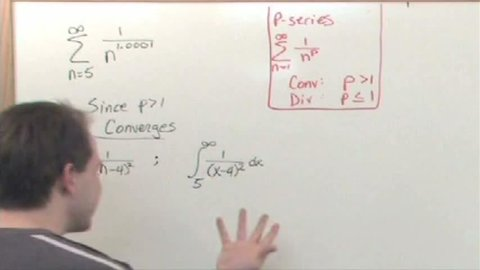# Integral Test of Series Convergence

##### TMW Media## Related videos

Alternating Series Test of Convergence
This program covers the important topic of Alternating Series Tests of Convergence in Calculus. We begin by discussing what an Alternating Series Test is and why it is a central topic in Calculus. Next, we solve several practical calculus problems that give students practice with these calculations. The entire lesson…
Ratio & Root Test of Series Convergence
This program covers the important topic of Ratio and Root Tests of Series Convergence in Calculus. We begin by discussing what a Ratio and Root Test is and why it is a central topic in Calculus. Next, we solve several practical calculus problems that give students practice with these calculations.…
Comparison Tests Of Series Convergence
This program covers the important topic of Comparison Tests of Series Convergence in Calculus. We begin by discussing what a Comparison Test is and why it is a central topic in Calculus. Next, we solve several practical calculus problems that give students practice with these calculations. The entire lesson is…
Trigonometric Integrals
This program covers the important topic of Trigonometric Integrals in Calculus. We begin by discussing what Trigonometric Integrals are and why it is a central topic in Calculus. Next, we solve several practical calculus problems that give students practice with Trigonometric Integrals. The entire lesson is taught by working example…
Series
This program covers the important topic of Series in Calculus. We begin by discussing what a Series is and why it is a central topic in Calculus. Next, we solve several practical calculus problems that give students practice with Series. The entire lesson is taught by working example problems beginning…
Inverse Trigonometric Functions
This program covers the important topic of Inverse Trigonometric Functions in Calculus. We begin by discussing what an inverse trigonometric function is and why it is a central topic in Calculus. Next, we solve several practical calculus problems that give students practice with inverse trigonometric functions. The entire lesson is…
Derivatives of Inverse Trigonometric Functions
This program covers the important topic of Derivatives of Inverse Trigonometric Functions in Calculus. We begin by discussing what an inverse trigonometric function is, how to take its derivative, and why it is a central topic in Calculus. Next, we solve several practical calculus problems that give students practice with…
Hyperbolic Functions
This program covers the important topic of Hyperbolic Functions in Calculus. We begin by discussing what a Hyperbolic function is and why it is a central topic in Calculus. Next, we solve several practical calculus problems that give students practice with Hyperbolic functions. The entire lesson is taught by working…
Inverse Hyperbolic Functions
This program covers the important topic of Inverse Hyperbolic Functions in Calculus. We begin by discussing what an Inverse Hyperbolic function is and why it is a central topic in Calculus. Next, we solve several practical calculus problems that give students practice with Inverse Hyperbolic functions. The entire lesson is…
L'Hospital's Rule
This program covers the important topic of L'Hospital's Rule in Calculus. We begin by discussing what L'Hospital's Rule is, how it is used to take derivatives, and why it is a central topic in Calculus. Next, we solve several practical calculus problems that give students practice with L'Hospital's Rule. The…
Integration By Partial Fractions
This program covers the important topic of Integration by Partial Fractions in Calculus. We begin by discussing what a Partial Fraction is, how it can be used to solve integrals, and why it is a central topic in Calculus. Next, we solve several practical calculus problems that give students practice…
Arc Length
This program covers the important topic of Arc Length in Calculus. We begin by discussing what Arc Length is and why it is a central topic in Calculus. Next, we solve several practical calculus problems that give students practice with Arc Length. The entire lesson is taught by working example…